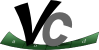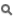Vc  1.1.0 SIMD Vector Classes for C++Detailed Description

Mask classes are abstractions for the results of vector comparisons. The actual implementation differs depending on the SIMD instruction set. On SSE they contain a full 128-bit datatype while on a different architecture they might be bit-fields.

Classes

The main SIMD mask class. More...

Functions

template<typename T , typename Abi >
std::ostream & operator<< (std::ostream &out, const Vc::Mask< T, Abi > &m)
Prints the contents of a mask into a stream object. More...

using double_m = Mask< double >

using float_m = Mask< float >

using llong_m = Mask< llong >

using ullong_m = Mask< ullong >

using long_m = Mask< long >

using ulong_m = Mask< ulong >

using int_m = Mask< int >

using uint_m = Mask< uint >

using short_m = Mask< short >

using ushort_m = Mask< ushort >

using schar_m = Mask< schar >

using uchar_m = Mask< uchar >

Function Documentation

 std::ostream& Vc::operator<< ( std::ostream & out, const Vc::Mask< T, Abi > & m )
inline

Prints the contents of a mask into a stream object.

std::cout << m << std::endl;

will output (with SSE):

m[1110 0000]
Parameters
 out Any standard C++ ostream object. For example std::cout or a std::stringstream object. m Any Vc::Mask object.
Returns
The ostream object: to chain multiple stream operations.
Note
With the GNU standard library this function will check whether the output stream is a tty in which case it colorizes the output.

Definition at line 158 of file IO.